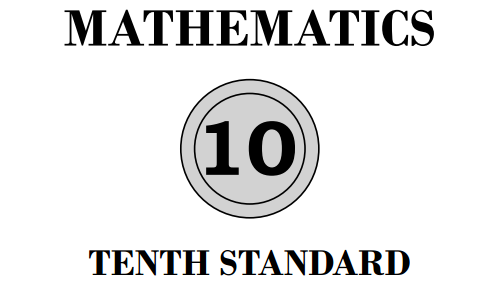# KSEEB SSLC Class 10 Maths Solutions Karnataka State Syllabus

Students who are studying to learn 10th Maths can go with this page. On this page, you will find the Chapterwise Pdf links of Karnataka Board for KSEEB solutions for class 10 maths. By just accessing the respective chapter link, you will be able to learn KTBS Karnataka State for 10th Standard Textbooks Solutions Answers Guide, Notes Pdf, and also practise chapter wise exercises in an easy manner. So, we would suggest you to download the KSEEB SSLC Class 10 Solutions for Maths PDF & gain good marks.

## Karnataka State Board Syllabus for Class 10 Maths Solutions

Textbook learning is most important for the students while exam preparation. All chapters are explained very conceptually in the textbook so students are recommended to learn all the concepts covered in the 10 Maths via KTBS Solutions. You will find all questions and answers, notes, exercises for all chapters in KSEEB SSLC Class 10 Maths Solutions Karnataka State Syllabus PDF. So get them and prepare properly for the final exams. Students can also read Karnataka SSLC Maths Model Question Papers with Answers 2020-2021 Pdf.10th Standard Maths Textbook Solutions Karnataka State Syllabus in English Medium

KSEEB Solutions For Class 10 Maths Chapter 1 Arithmetic Progressions

KSEEB 10th Maths Solutions Chapter 2 Triangles

SSLC Maths Solutions Class 10 KSEEB Chapter 3 Pair of Linear Equations in Two Variables

10th Standard Karnataka State Syllabus Maths Guide Chapter 4 Circles

Karnataka State Board 10th Maths Solutions Chapter 5 Areas Related to Circles

SSLC Maths Solutions KSEEB Chapter 6 Constructions

10th Maths In Kannada Solutions for Class 10 Chapter 7 Coordinate Geometry

KSEEB SSLC Class 10 Maths Solutions Chapter 8 Real Numbers

10th Maths Syllabus State Board Karnataka Solutions Chapter 9 Polynomials

SSLC Mathematics Solution Part 2 KSEEB Chapter 10 Quadratic Equations

Maths Guide For Class 10 State Syllabus Karnataka Chapter 11 Introduction to Trigonometry

Maths Solutions for Class 10 State Syllabus Karnataka Chapter 12 Some Applications of Trigonometry

Karnataka SSLC Maths Text Book Solutions Chapter 13 Statistics

Karnataka State Board Syllabus for Class 10 Maths textbook Solutions Chapter 14 Probability

Karnataka SSLC Maths Notes Pdf Chapter 15 Surface Areas and Volumes

## Karnataka State Board Syllabus for Class 10 Maths Solutions in Kannada Medium

10th Standard Maths Textbook Solutions Karnataka State Syllabus in Kannada Medium

KSEEB Solutions For Class 10 Maths Chapter 1 Arithmetic Progressions

KSEEB 10th Maths Solutions Chapter 2 Triangles

SSLC Maths Solutions Class 10 KSEEB Chapter 3 Pair of Linear Equations in Two Variables

10th Standard Karnataka State Syllabus Maths Guide Chapter 4 Circles

Karnataka State Board 10th Maths Solutions Chapter 5 Areas Related to Circles

SSLC Maths Solutions KSEEB Chapter 6 Constructions

10th Maths In Kannada Solutions for Class 10 Chapter 7 Coordinate Geometry

KSEEB SSLC Class 10 Maths Solutions Chapter 8 Real Numbers

10th Maths Syllabus State Board Karnataka Solutions Chapter 9 Polynomials

SSLC Mathematics Solution Part 2 KSEEB Chapter 10 Quadratic Equations

Maths Guide For Class 10 State Syllabus Karnataka Chapter 11 Introduction to Trigonometry

Maths Solutions for Class 10 State Syllabus Karnataka Chapter 12 Some Applications of Trigonometry

Karnataka SSLC Maths Text Book Solutions Chapter 13 Statistics

Karnataka State Board Syllabus for Class 10 Maths textbook Solutions Chapter 14 Probability

Karnataka SSLC Maths Notes Pdf Chapter 15 Surface Areas and Volumes

If you need some more information about Karnataka State Board Solutions for 10th Maths then visit our site frequently and get all the resources that you look for. Also, share this page with your friends and make them learn all the concepts thoroughly before the exams.